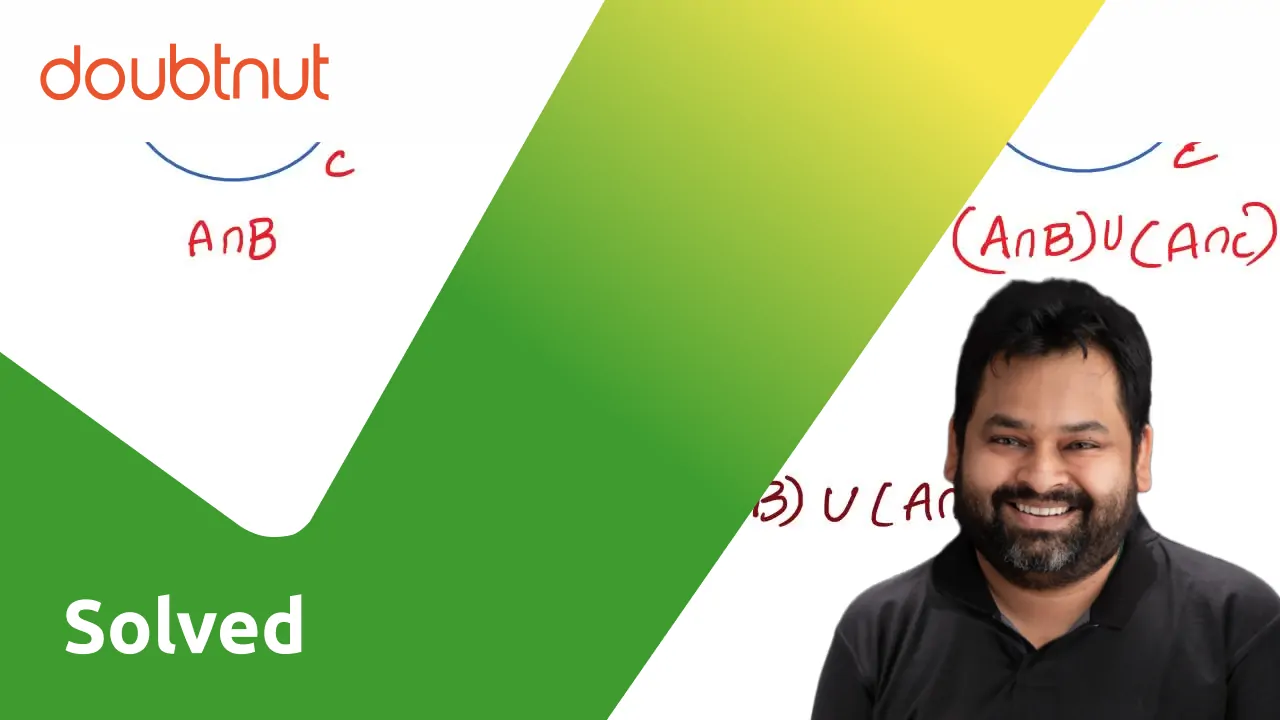Doubtnut App - Get 50 Lakh+ video solutions better than GoogleInstall Now

# Using Venn diagram, prove the following i. A∪(B∩C)=(A∪B)∩(A∪C) ii. A∩(B∪C)=(A∩B)∪(A∩C)Solution in Tamil

Text Solution
Verified by Experts

## The correct Answer is:From the figures (b) and (e), we get A∩(B∪C)=(A∩B)∪(A∩C)

Video Solution